### ISBLANK Function Examples – Excel & Google Sheets

This tutorial demonstrates how to use the Excel ISBLANK Function in Excel to test if a cell is blank.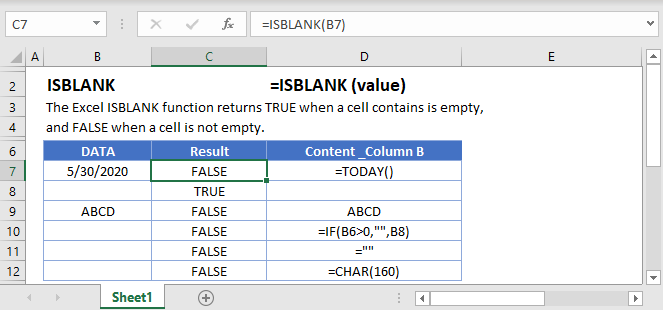## ISBLANK Function Overview

The ISBLANK Function Test if cell is blank. Returns TRUE or FALSE.

To use the ISBLANK Excel Worksheet Function, select a cell and type: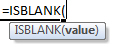(Notice how the formula inputs appear)

### ISBLANK Function Syntax and Inputs:

`=ISBLANK(VALUE)`

value – The test value

AutoMacro - VBA Code Generator

## How to use the ISBLANK Function

The ISBLANK Function tests if a cell is completely blank or not. It returns TRUE if the cell is blank, FALSE otherwise.

`=ISBLANK(A2)`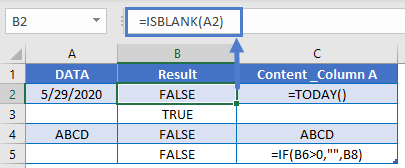### If Cell is Blank Then

Often, you’ll want to combine an “IS” function, like ISBLANK, with the IF Function. With the IF Function, instead of returning a simple TRUE or FALSE, you can output specific text or perform specific actions if a cell is blank or not.

`=IF(ISBLANK(C2),"",C2*D2)`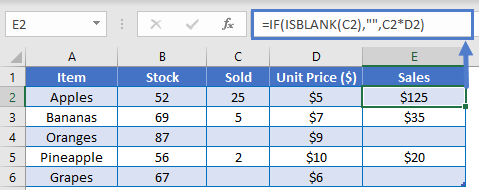### IF Cell is Not Blank Then

With the NOT Function, you can test the inverse: is a cell not blank?

`=IF(NOT(ISBLANK(C2)),C2*D2,"")`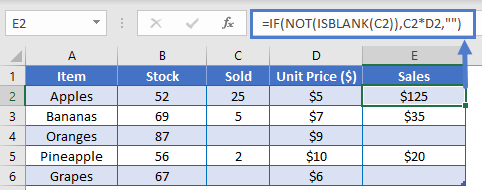### ISBLANK Range – COUNTBLANK

To test a range for blank values, you could use the ISBLANK Function along with the SUMPRODUCT Function. However, the easiest way to test for blank cells is with the COUNTBLANK Function.

The COUNTBLANK Function counts the number of blank cells in a range.

`=COUNTBLANK(A2:C7)`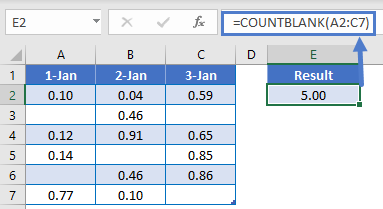If COUNTBLANK > 0 then there is at least one blank cell in the range. Use this along with an IF Statement to do something if a blank cell is found.

`=IF(COUNTBLANK(A2:C2)>0,100*SUM(A2:C2),200*SUM(A2:C2))`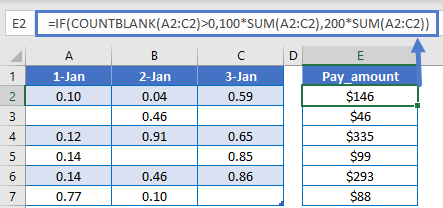### ISBLANK Function Not Working?

The ISBLANK Function will return FALSE for cells that look blank, but are not. Including:

• Zero-length string from an external data source
• spaces, apostrophes, non-breaking spaces (&nbsp;), or other non-printing characters.
• An empty string (“”)

By using IF and OR functions you can identify cells with empty strings as well as normal blank cells.

`=IF(OR(ISBLANK(A2),A2=""),"blank","not blank")`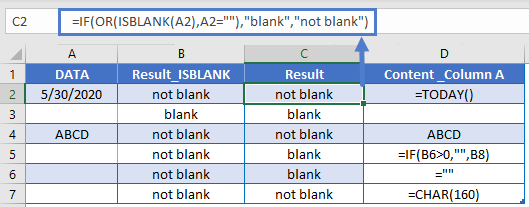Another option is to use the TRIM Function to trim off extra spaces and the LEN Function to count the number of characters.

`=IF(OR(A2=" ", LEN(TRIM(A2))=0), "blank", "not blank")`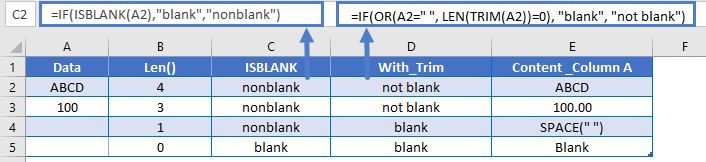### Other Logical Functions

Excel / Google Sheets contain many other logical functions to perform other logical tests. Here is a list:

IF / IS Functions
iferror
iserror
isna
iserr
isblank
isnumber
istext
isnontext
isformula
islogical
isref
iseven
isodd

## ISBLANK in Google Sheets

The ISBLANK Function works exactly the same in Google Sheets as in Excel: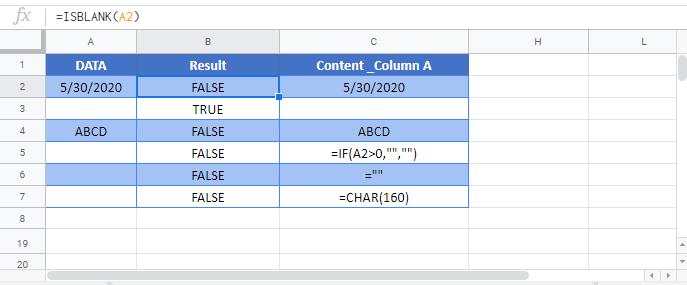## Excel Practice Worksheet

Practice Excel functions and formulas with our 100% free practice worksheets!

• Automatically Graded Exercises
• Learn Excel, Inside Excel!